# 2. [-75 Points] DETAILS SCALCCC4 7.5.007.MI. MY NOTES ASK YOUR TEACHER PRACTICE ANOTHER The population of...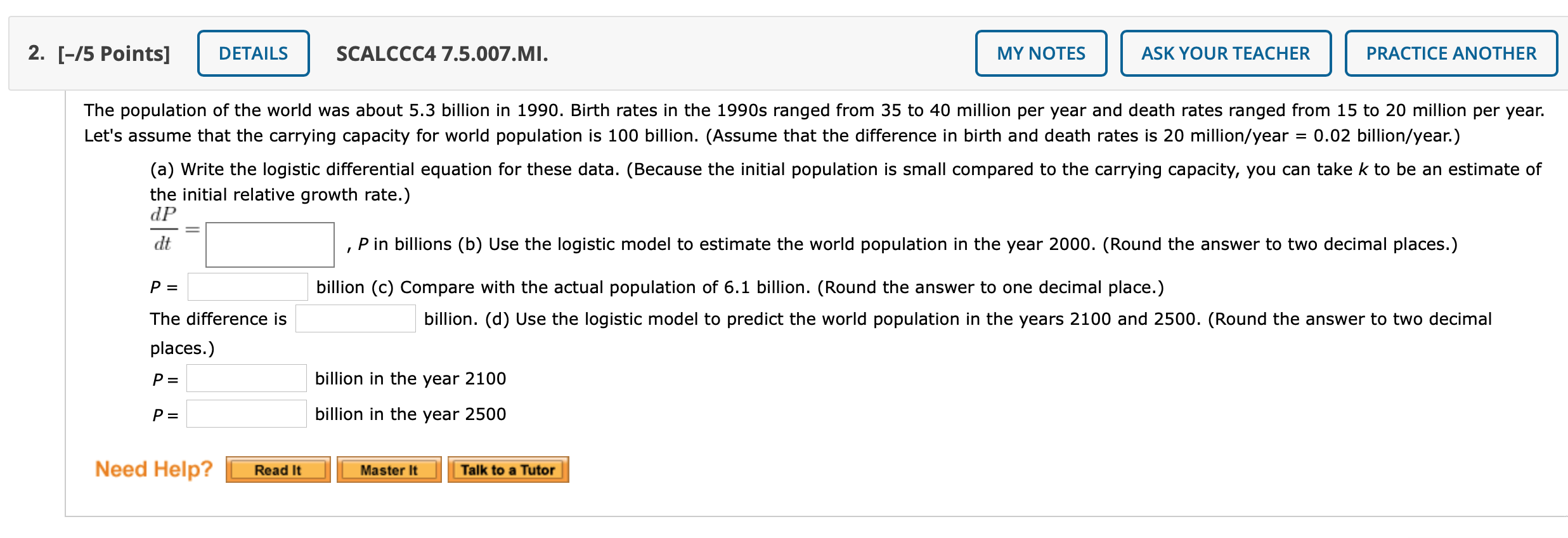2. [-75 Points] DETAILS SCALCCC4 7.5.007.MI. MY NOTES ASK YOUR TEACHER PRACTICE ANOTHER The population of the world was about 5.3 billion in 1990. Birth rates in the 1990s ranged from 35 to 40 million per year and death rates ranged from 15 to 20 million per year. Let's assume that the carrying capacity for world population is 100 billion. (Assume that the difference in birth and death rates is 20 million/year 0.02 billion/year.) (a) Write the logistic differential equation for these data. (Because the initial population is small compared to the carrying capacity, you can take k to be an estimate of the initial relative growth rate.) dP dt , P in billions (b) Use the logistic model to estimate the world population in the year 2000. (Round the answer to two decimal places.) P= billion (c) Compare with the actual population of 6.1 billion. (Round the answer to one decimal place.) billion. (d) Use the logistic model to predict the world population in the years 2100 and 2500. (Round the answer to two decimal The difference is places.) P= billion in the year 2100 P= billion in the year 2500 Need Help? Read It Master It Talk to a Tutor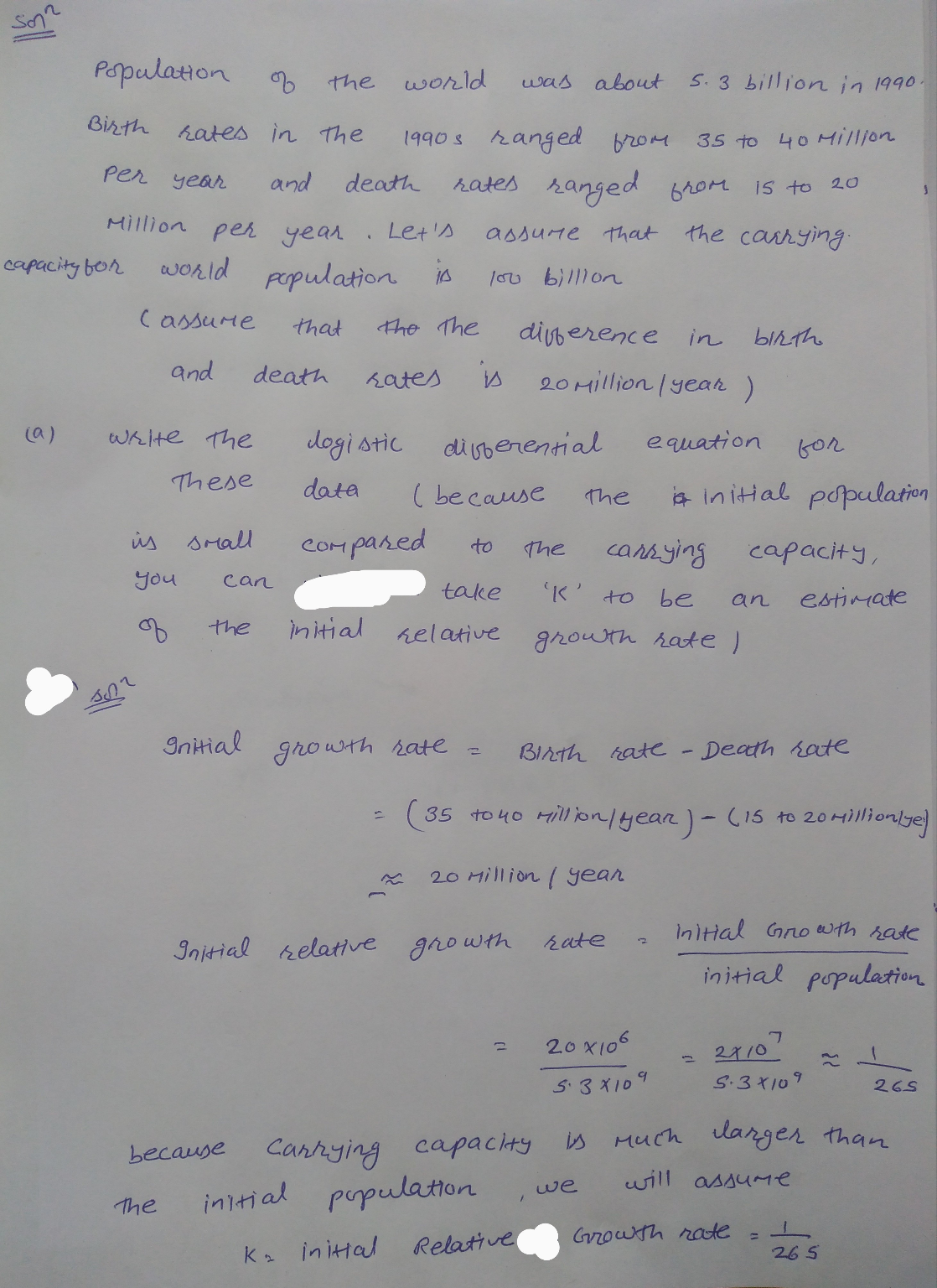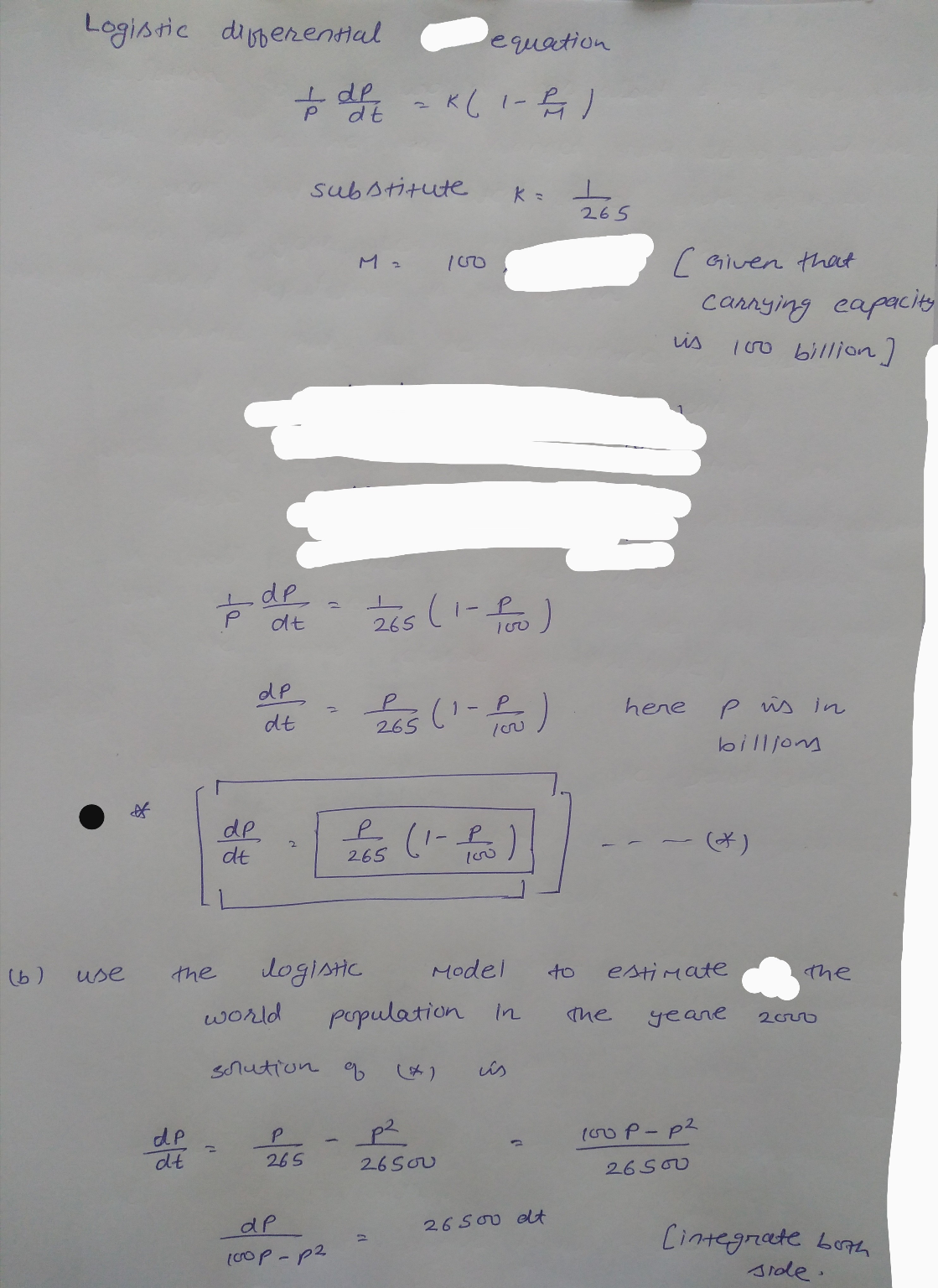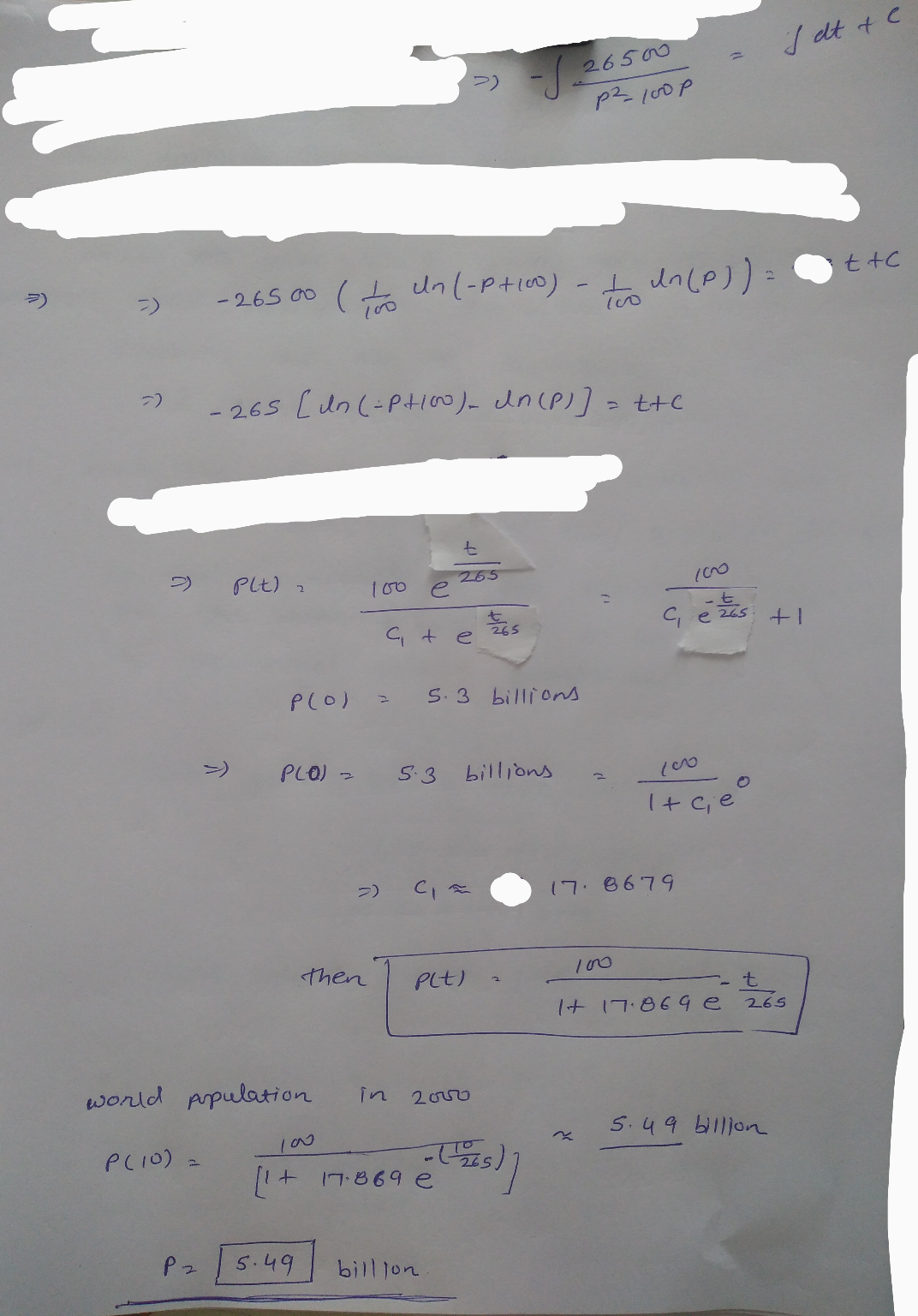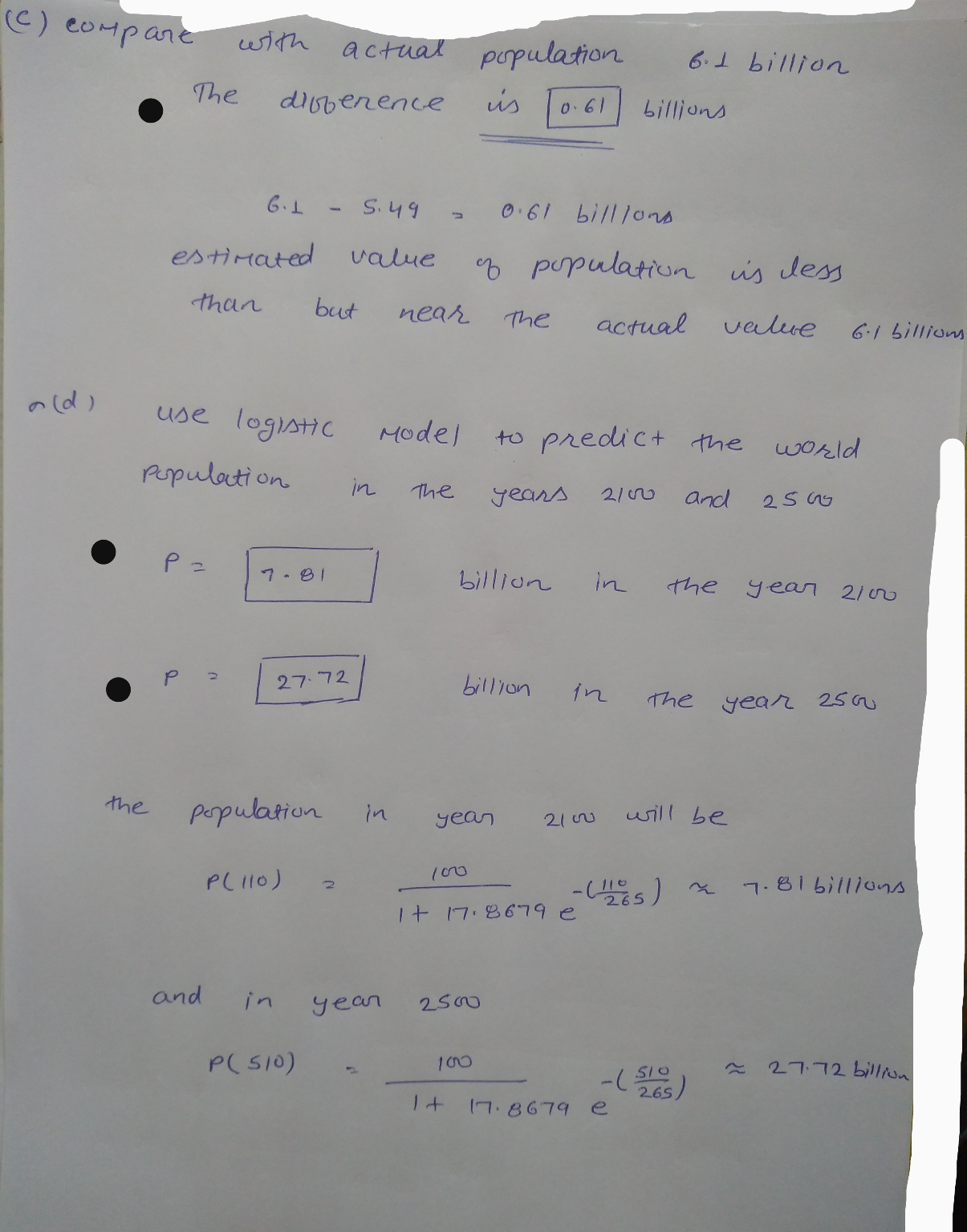Similar Homework Help Questions
• ### 2. [0/5 Points] DETAILS PREVIOUS ANSWERS SCALCCC4 7.5.007.MI. MY NOTES ASK YOUR TEACHER PRACTICE ANOTHER The...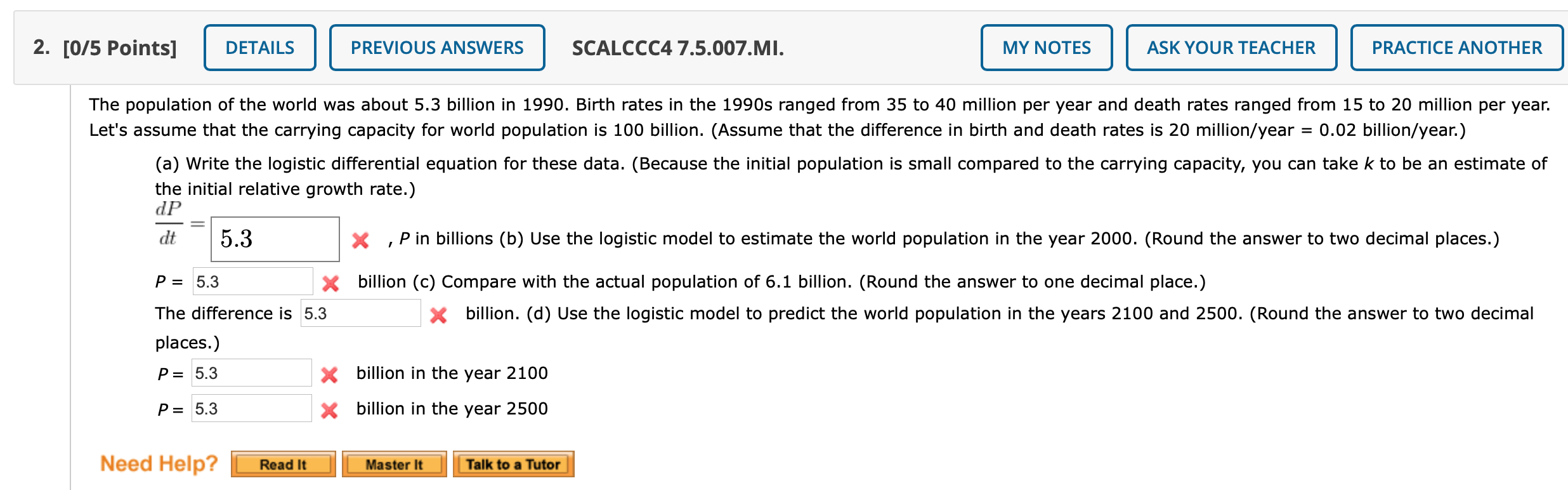2. [0/5 Points] DETAILS PREVIOUS ANSWERS SCALCCC4 7.5.007.MI. MY NOTES ASK YOUR TEACHER PRACTICE ANOTHER The population of the world was about 5.3 billion in 1990. Birth rates in the 1990s ranged from 35 to 40 million per year and death rates ranged from 15 to 20 million per year. Let's assume that the carrying capacity for world population is 100 billion. (Assume that the difference in birth and death rates is 20 million/year 0.02 billion/year.) (a) Write the logistic...

• ### Hi, I'm stuck. HELP!!!!! 0/2.2 points 21. Previous Answers SCalcET8 9.4.501.XPM My Notes Ask Your Teacher...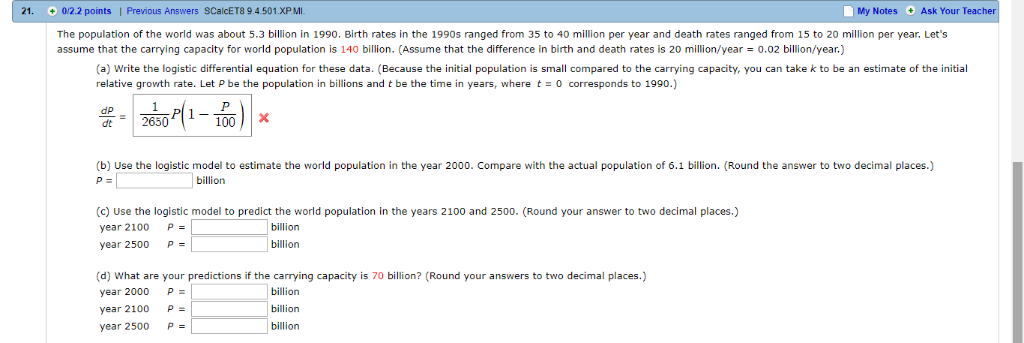Hi, I'm stuck. HELP!!!!! 0/2.2 points 21. Previous Answers SCalcET8 9.4.501.XPM My Notes Ask Your Teacher The population assume that the carrying capacity for world population is 140 billion. (Assume that the difference in birth and death rates is 20 million/year f the world was about 5.3 billion in 1990. Birth rates in the 1990s ranged from 35 to 40 million per year and death rates ranged from 15 to 20 million per year. Let's 0.02 billion/year.) the initial (a)...

• ### 6. 0.2/1 points | Previous Answers SCalcET8 9.4.009 My Notes Ask Your Suppose the population of the world was about 6.4 billion in 2000. Birth rates around that time ranged from 35 to 40 million per...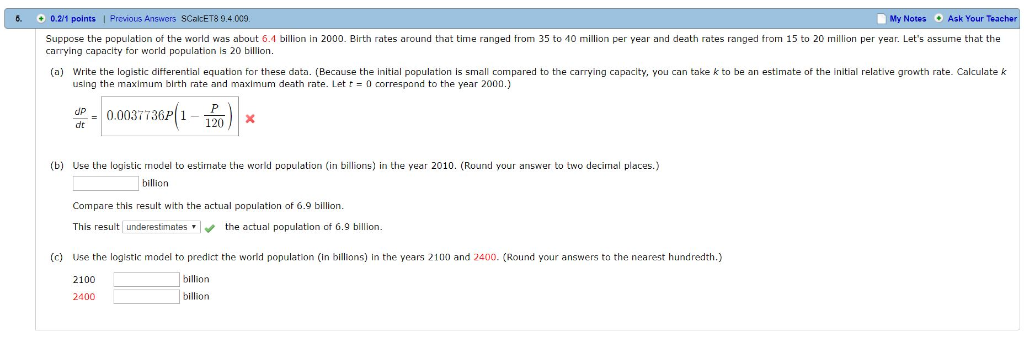6. 0.2/1 points | Previous Answers SCalcET8 9.4.009 My Notes Ask Your Suppose the population of the world was about 6.4 billion in 2000. Birth rates around that time ranged from 35 to 40 million per year and death rates ranged from 15 to 20 million per year. Let's assume that the carrying capacity for world population is 20 billion (a) Write the logistic differential equation for these data. (Because the initial population is small compared to the carrying capacity,...

• ### 1, 5 and 6 please! 1. (-/1 Points) DETAILS OSCOLALG16.7.380. MY NOT ASK YOUR TEACHER PRACTICE...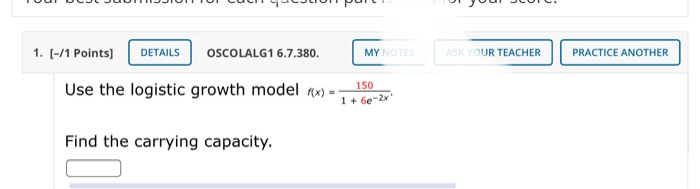1, 5 and 6 please! 1. (-/1 Points) DETAILS OSCOLALG16.7.380. MY NOT ASK YOUR TEACHER PRACTICE ANOTHER 150 Use the logistic growth model RX) - 1 + 6e-2x Find the carrying capacity. 5. (-/1 Points) DETAILS OSCOLALG1 6.7.392. MY NOTES ASK YOUR TEACHER PRACTICE ANOTHER Use a graphing calculator and the following scenario. The population P of a fish farm in t years is modeled by the equation PCC) 1200 1 + 9e-0.77 To the nearest whole number, what will...

• ### 6. (-/2 Points) DETAILS SCALCCC4 1.7.029. MY NOTES ASK YOUR TEACHER PRACTICE ANOTHER The parametric equations...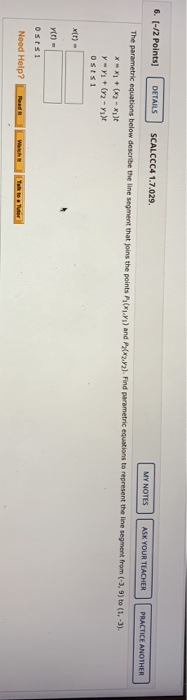6. (-/2 Points) DETAILS SCALCCC4 1.7.029. MY NOTES ASK YOUR TEACHER PRACTICE ANOTHER The parametric equations below describe the line segment that joins the points P) and P202.12). Find parametric equations to represent the line segment from (-3,9) to (1.-3). ***1 + (x2 - 1) ya Yu + (y2 - Yut Osts 1 Yt) Ostsi Nood Help? Wh

• ### 4. (-/3 Points] DETAILS SCALCCC4 6.5.010. MY NOTES ASK YOUR TEACHER PRACTICE ANOTHER Consider the given...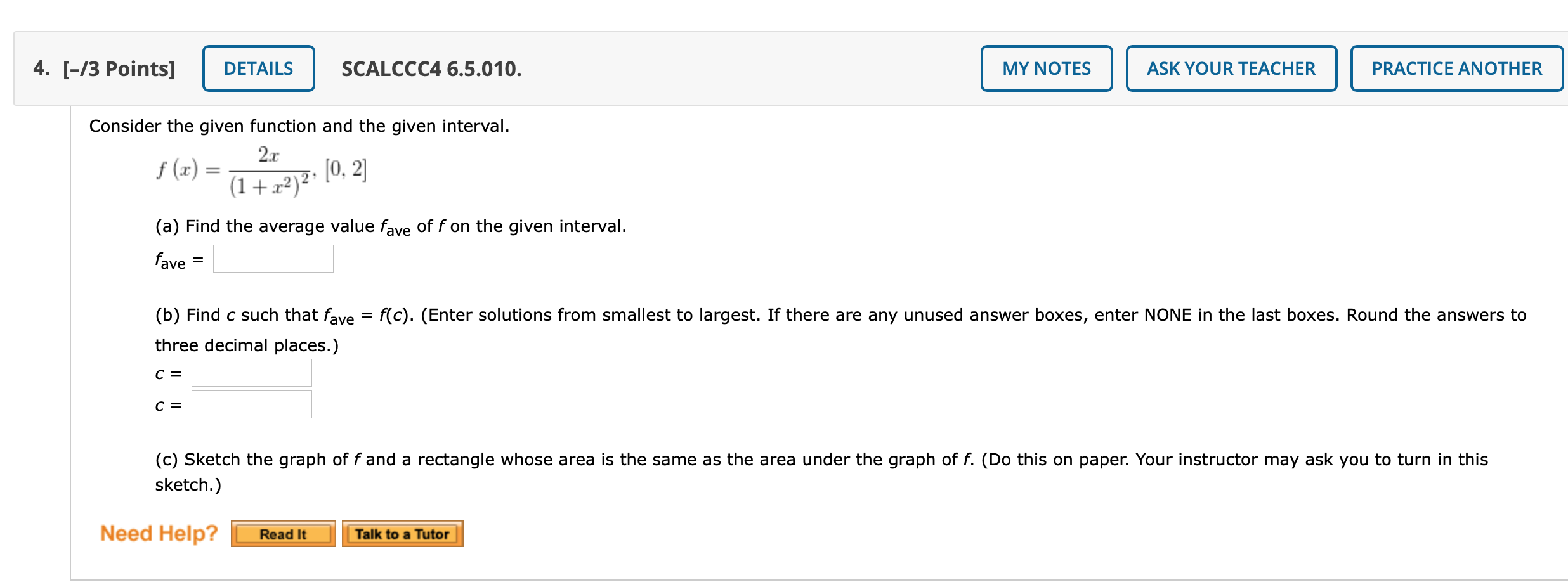4. (-/3 Points] DETAILS SCALCCC4 6.5.010. MY NOTES ASK YOUR TEACHER PRACTICE ANOTHER Consider the given function and the given interval. 2.r f (0) [0, 2] (1+x2) (a) Find the average value fave of f on the given interval. fave (b) Find c such that fave = f(c). (Enter solutions from smallest to largest. If there are any unused answer boxes, enter NONE in the last boxes. Round the answers to three decimal places.) C= C = (c) Sketch the...

• ### 9. (-/4 Points DETAILS JMODD8 4.4.016 MY NOTES ASK YOUR TEACHER PRACTICE ANOTHER A population X...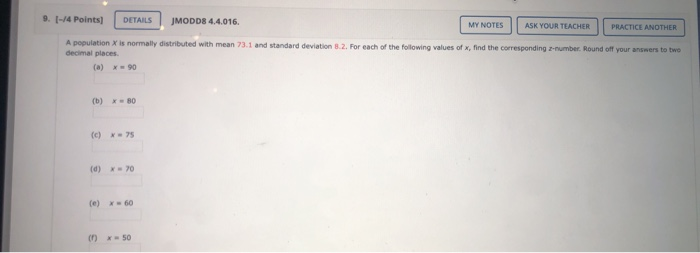9. (-/4 Points DETAILS JMODD8 4.4.016 MY NOTES ASK YOUR TEACHER PRACTICE ANOTHER A population X is normally distributed with mean 73.1 and standard deviation 8.2. For each of the following values of x, find the corresponding 2-number. Round off your answers to two decimal places (a) X 90 (b) x=80 (c) - 75 (d) x 70 (e) = 60 50 10. (-/4 Points] DETAILS JMODD8 4.4.017.CMI. MY NOTES ASK YOUR TEACHER PRACTICE ANO A population is normally distributed with...

• ### 1/2 Points) DETAILS PREVIOUS ANSWERS SALGTRIG4 4.6.007.MI. MY NOTES ASK YOUR TEACHER This exercise uses the...1/2 Points) DETAILS PREVIOUS ANSWERS SALGTRIG4 4.6.007.MI. MY NOTES ASK YOUR TEACHER This exercise uses the population growth model The population of a country has a relative growth rate of 4% per year. The government is trying to reduce the growth rate to 3%. The population in 2011 was approximately 120 million. Find the projected population for the year 2040 for the following conditions. (Round your answers to the nearest whole number.) () The relative growth rate remains at 4...

• ### 2. [0/1 Points) DETAILS PREVIOUS ANSWERS TANAPCALC10 5.6.003. MY NOTES ASK YOUR TEACHER PRACTICE ANOTHER Growth...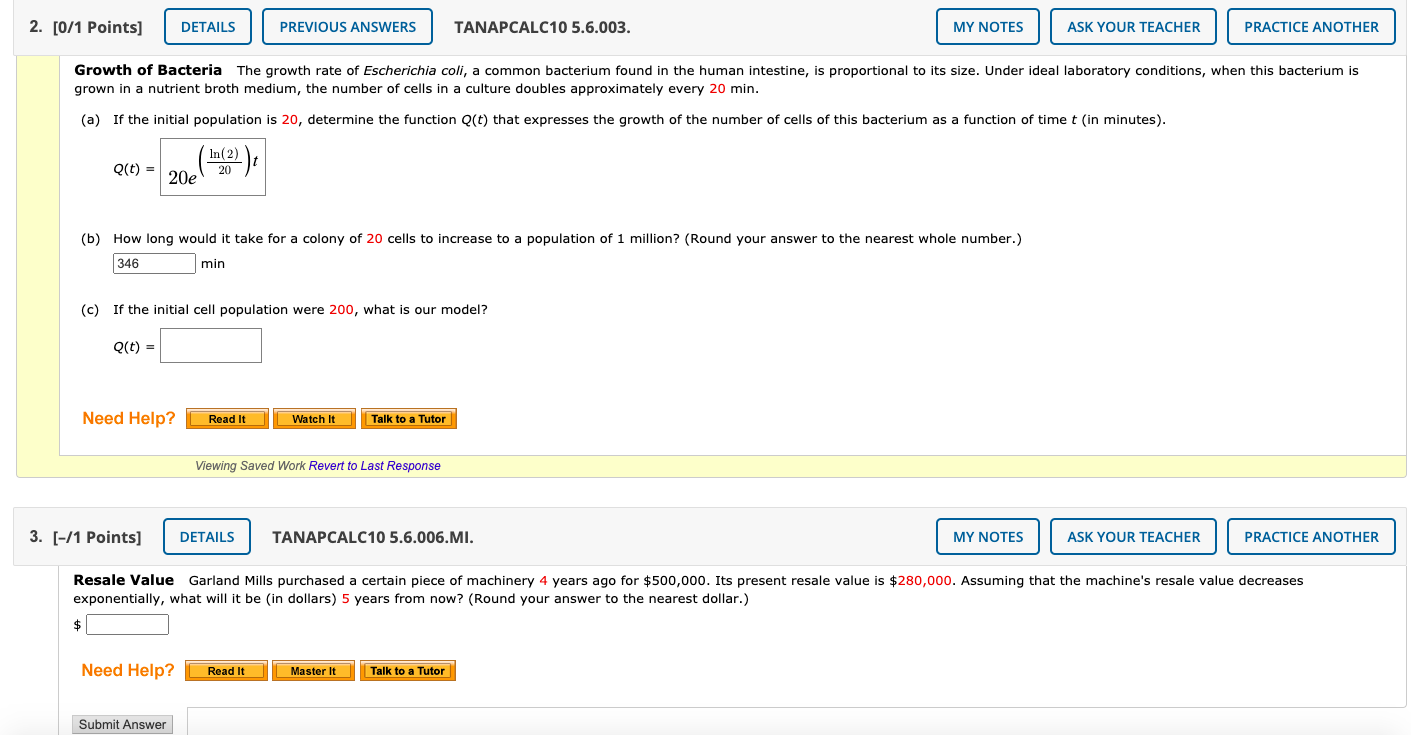2. [0/1 Points) DETAILS PREVIOUS ANSWERS TANAPCALC10 5.6.003. MY NOTES ASK YOUR TEACHER PRACTICE ANOTHER Growth of Bacteria The growth rate of Escherichia coli, a common bacterium found in the human intestine, is proportional to its size. Under ideal laboratory conditions, when this bacterium is grown in a nutrient broth medium, the number of cells in a culture doubles approximately every 20 min. (a) If the initial population is 20, determine the function Q(t) that expresses the growth of the...

• ### webassign.net 4. (-/10.71 Points) DETAILS BBUNDERSTAT128.5.013.MI. MY NOTES ASK YOUR TEACHER PRACTICE ANOTHER For one binomial...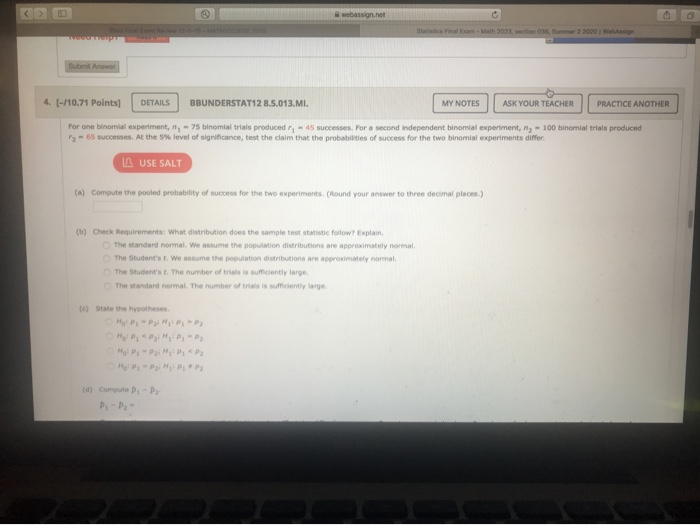webassign.net 4. (-/10.71 Points) DETAILS BBUNDERSTAT128.5.013.MI. MY NOTES ASK YOUR TEACHER PRACTICE ANOTHER For one binomial experiment, , - 75 binomial trials produced ry - 45 successes. For a second Independent binomial experiment, ', - 100 binomial trials produced 2 - 65 successes. At the 5% level of significance, test the claim that the probabilities of success for the two binomial experiments differ. USE SALT (a) Compute the pooled probability of success for the two experiments. (Round your answer to...

Free Homework App